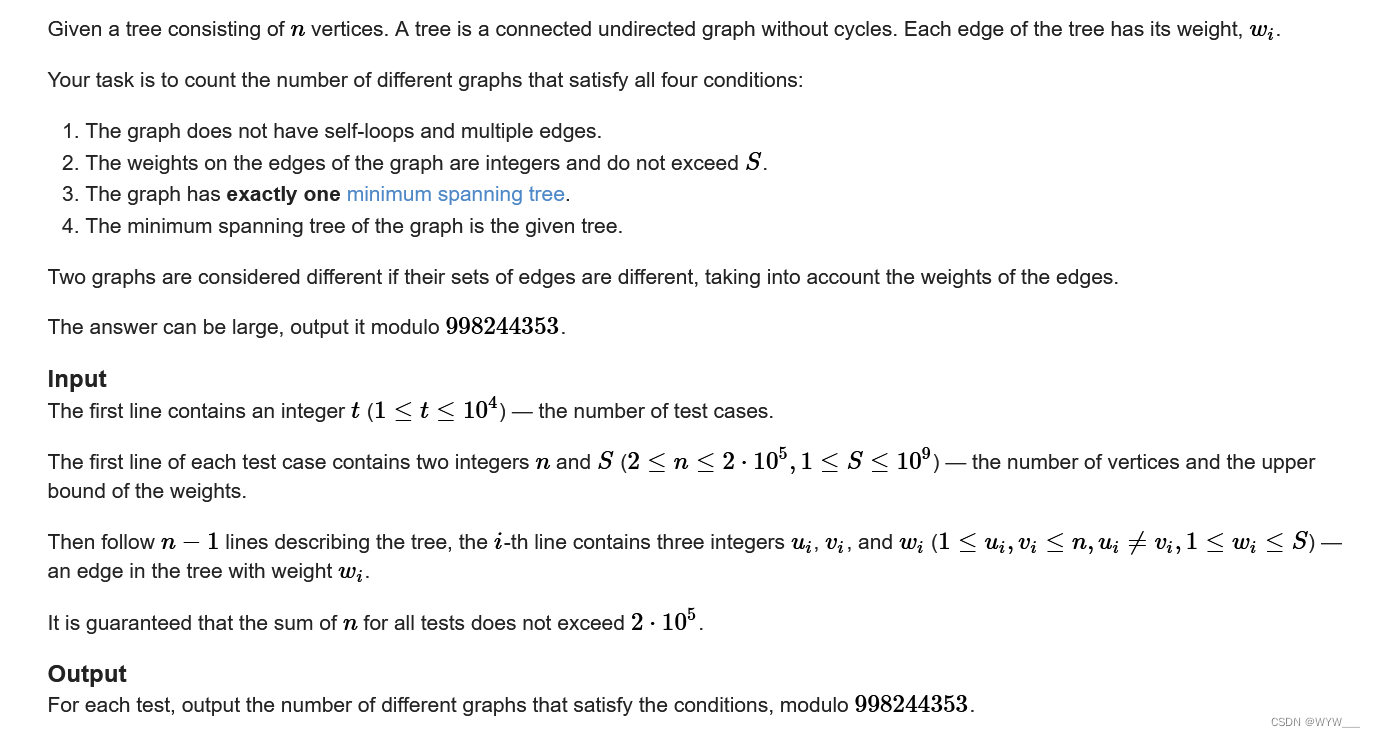# G. Counting Graphs(并查集)

Problem - G - CodeforcesPlain Text

图形没有自环和多重边。



Example

Input

Copy

4

2 5

1 2 4

4 5

1 2 2

2 3 4

3 4 3

5 6

1 2 3

1 3 2

3 4 6

3 5 1

10 200

1 2 3

2 3 33

3 4 200

1 5 132

5 6 1

5 7 29

7 8 187

7 9 20

7 10 4

Output

Copy

1
8
80
650867886


#include<bits/stdc++.h>
using namespace std;
#define int long long
struct node
{
int x,y,w;
}a;
bool cmp(node a,node b)
{
return a.w < b.w;
}
int f,d;
int find(int x)
{
if(f[x] == x)
return f[x];
return f[x] = find(f[x]);
}
int mod = 998244353;
int qpow(int x,int p)
{
int ans = 1;
while(p)
{
if(p&1)
ans = ans*x%mod;
x = x*x%mod;
p /= 2;
}
return ans;
}
void solve()
{
int n,s;
cin >> n >> s;
for(int i = 1;i < n;i++)
{
int x,y,w;
cin >> x >> y >> w;
a[i] = {x,y,w};
}
for(int i = 1;i <= n;i++)
{
f[i] = i;
d[i] = 1;
}
sort(a + 1,a + n,cmp);
int ans = 1;
for(int i = 1;i < n;i++)
{
int x = find(a[i].x);
int y = find(a[i].y);
ans = ans*qpow(s - a[i].w + 1,d[x]*d[y] - 1)%mod;
f[y] = x;
d[x] += d[y];
}
cout << ans <<"\n";
}
signed main()
{
int t = 1;
ios::sync_with_stdio(0);
cin.tie(0);
cout.tie(0);
cin >> t;
while(t--)
{
solve();
}
}

04-08
12-01

### “相关推荐”对你有帮助么？

•非常没帮助
•没帮助
•一般
•有帮助
•非常有帮助被折叠的  条评论 为什么被折叠?到【灌水乐园】发言WYW___

¥1 ¥2 ¥4 ¥6 ¥10 ¥20获取中扫码支付点击重新获取扫码支付1.余额是钱包充值的虚拟货币，按照1:1的比例进行支付金额的抵扣。
2.余额无法直接购买下载，可以购买VIP、付费专栏及课程。余额充值